## 《Objective-C高级编程：iOS与OS X多线程和内存管理》 一 Blocks模式GCD

el/2023/9/24 21:01:07

## 一、Blocks摘要

Blocks：带有自动变量（局部变量）的匿名函数。

``````int func(int cout); //声明名称为func的函数
int result = func(10); // 调用该函数，必须使用该函数的名称
// 若像下面这样，使用函数指针来代替直接调用函数，那么不知道函数名也可调用
int result = (*funptr)(10);
// 但使用函数指针也仍需知道函数名称，在赋值给函数指针时，若不使用想赋值的函数的名称，就无法取得该函数的地址。
int (*funcptr)(int) = &func;
int result = (* funcptr)(10);``````

## 2.1 Block 语法

^ 返回值类型 参数列表 表达式

``````^(int count）{return count+1;} //按照return 语句的类型，返回int型返回值
^void (void){print("Blocks\n");}//参数列表省略
^{print("Blocks\n");}//返回值类型、参数列表都省略``````

## 2.2 Block类型变量

``````int func(int count）「return count+1;
}
int (*funcptr)(int) = &func;
``````

• 函数指针变量：指向函数入口的指针变量(本质是变量)
• 类型类型说明符 (*指针变量名)(形参表);          int　(*function)(int i);
• 函数指针变量：指向函数入口的指针变量(本质是变量)
• 类型说明符 *函数名(形参表){

}  int  *function(int i){}

``int（^blk)(int);``

``int (^blk)(int) = ^(int count){return count+1;};``

``````int（^blk1)(int) = blk;
int (^blk2)(int);
blk2 = blk1;``````

``````void func(int (^blk)(int)){
// 在函数返回值中指定Block类型，可以将Block做我饿函数的返回值返回int (^func()(int)){return ^(int count){return count+1;};}
}``````

``typedef int(^blk_int)(int)``

``````int result =（*funptr)(10);
int result = blk(10);``````

``````int func（blk_t blk, int rate){return blk(rate);
}
====
- (int) methodUsingBlock:(blk_t)blk rate:(int)rate{return blk(rate);
}``````

Block类型变量可完全像通常的C语言变量一样使用，因此也可以使用指向Block类型变量的指针，即Block的指针类型变量。

``````typedef int (^blk_t)(int);  //定义一个参数为int 返回值为int 的Block变量blk_t
blk_t blk = ^(int count){return count +1;};
blk_t *blkptr = &blk;
(*blkptr)(10);``````

## 2.3 截获自动变量值

https://www.jianshu.com/p/43f1058432e8

``````int main(int argc, const char * argv[]) {@autoreleasepool {int value0 = 1;int value1 = 2;        void (^myBlock)(void) = ^{NSLog(@"value0:%d   value1:%d", value0, value1);};        value0 = 10;value1 = 20;        myBlock();   // value0:1   value1:2}return 0;
}``````

## 2.4 __block说明符

``````int val = 0；
void (^blk)(void) = ^{val = 1};
blk();
printf("val = %d\n",val)；``````

``````_block int val = 0；
void (^blk)(void) = ^{val = 1};
blk();
printf("val = %d\n",val)；//val = 1``````

## 2.5 截获的自动变量

``````int val = 0;
void (^blk)(void) = ^{val =1;};``````

``````id array = [[NSMUtable allov] init];
void (^blk)(void) = ^{id obj = [[NSObjectArray alloc]init];[array addObject:obj];}
``````

``````id array = [[NSMUtable allov] init];
void (^blk)(void) = ^{array = [[NSObjectArray alloc]init];}
``````

``````_block id array = [[NSMUtable allov] init];
void (^blk)(void) = ^{array = [[NSObjectArray alloc]init];}
``````

``````const cha text[] = "hello";
void (^blk)(void) = ^{printf("%c\n",text);};``````

``````const char *text = "hello";
void (^blk)(void) = ^{printf("%c\n",text);
}``````

## 4.1 GCD摘要

### 4.1.1 GCD

``````dispatch_async (queue, ^{/*  长时间处理：例如：数据库访问，AR用画像识别 *//*  长时间处理结束，主线程使用该处理结束 */dispatch_async (dispatch_get_main_queue(), ^{/*  只在主线程可以执行的处理，例如用户界面更新 */}）；
}）；``````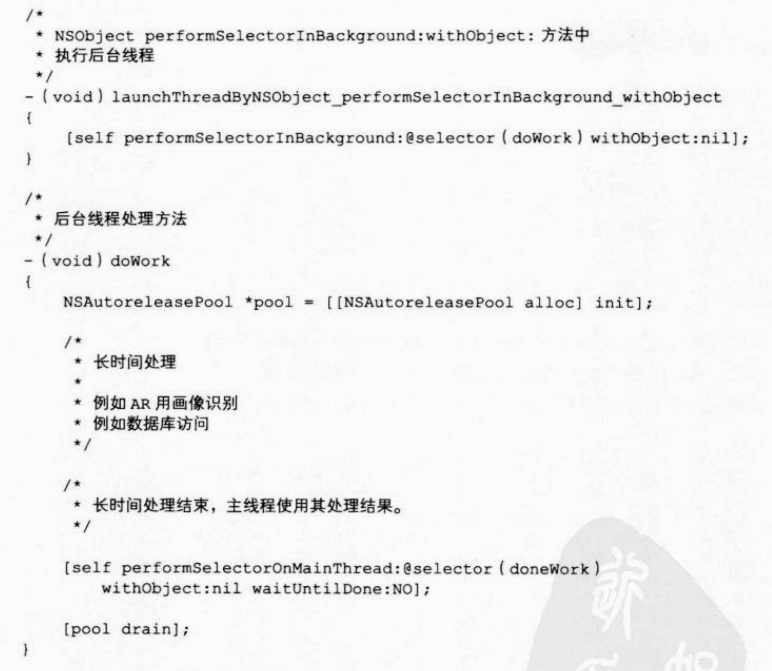``````// 主线程处理方法
- (void) donework{
//只有主线程可以执行的处理：例如：用户界面更新
}``````

### 4.1.2 多线程编程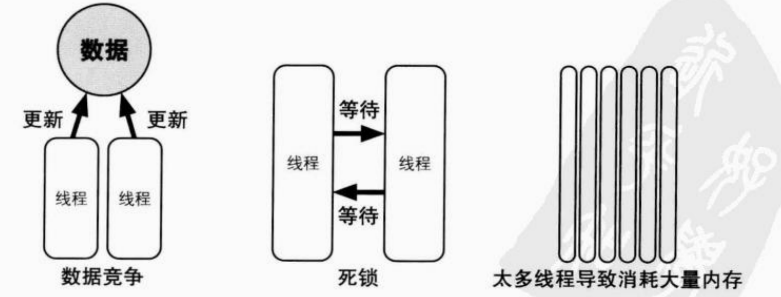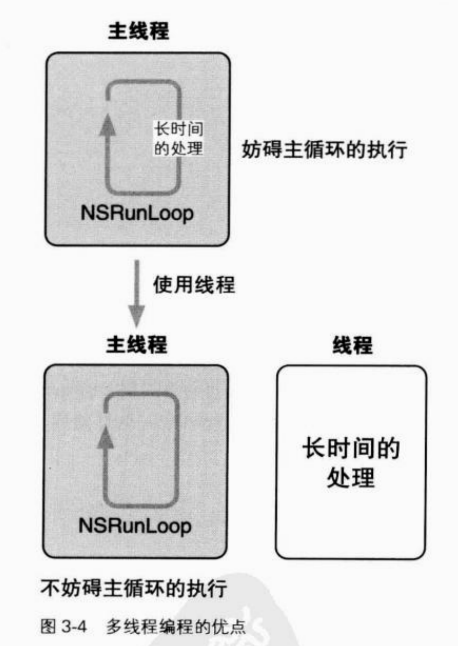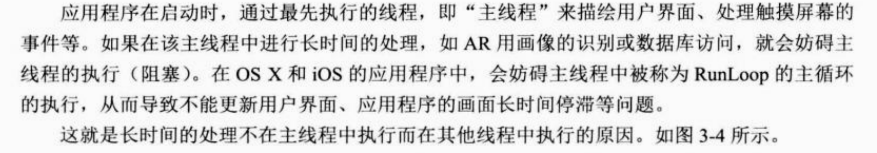## 4.2 GCD的API

### 4.2.1 Dispatch Queue

Dispatch Queue：是执行处理的等待队列，开发者通过此函数等API,在Block语法中记述想执行的处理并将其追加到Dispatch Queue中，Dispatch Queue按照追加的顺序（FIFO)执行处理。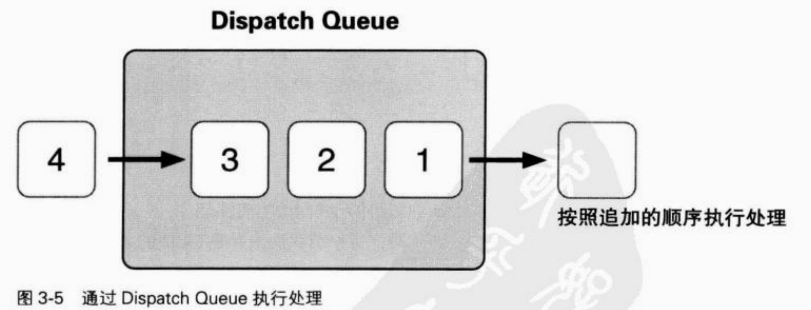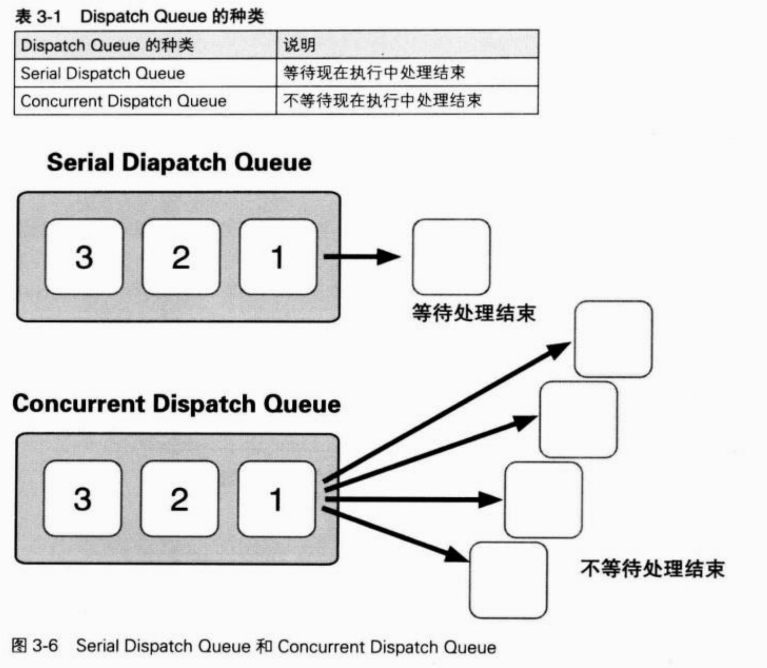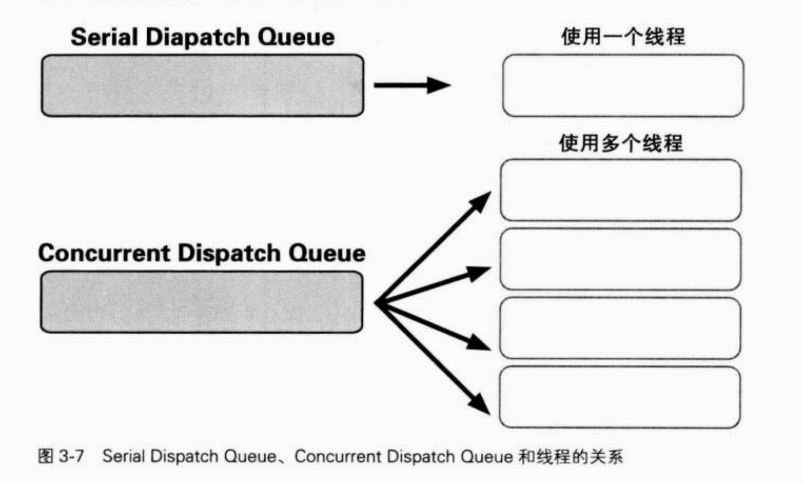XUN内核决定应当使用的线程数，并只生产所需的线程执行处理。当处理结束，应当执行的处理数减少时，XUN内核会结束不再需要的线程。XUN内核仅使用Concurrent Dispatch Queue 便可完美地管理并执行多个处理的线程。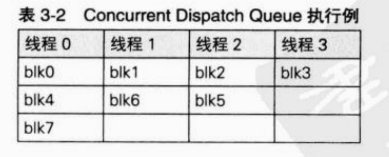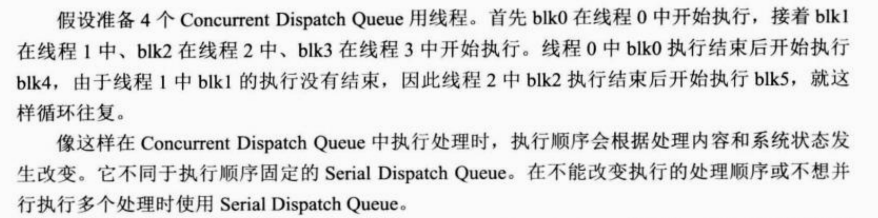### 4.2.2 dispatch_queue_create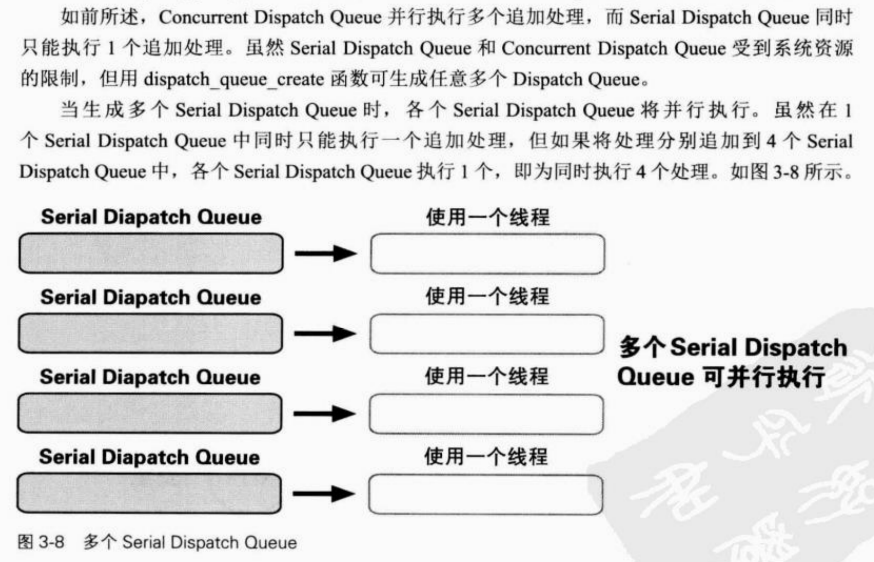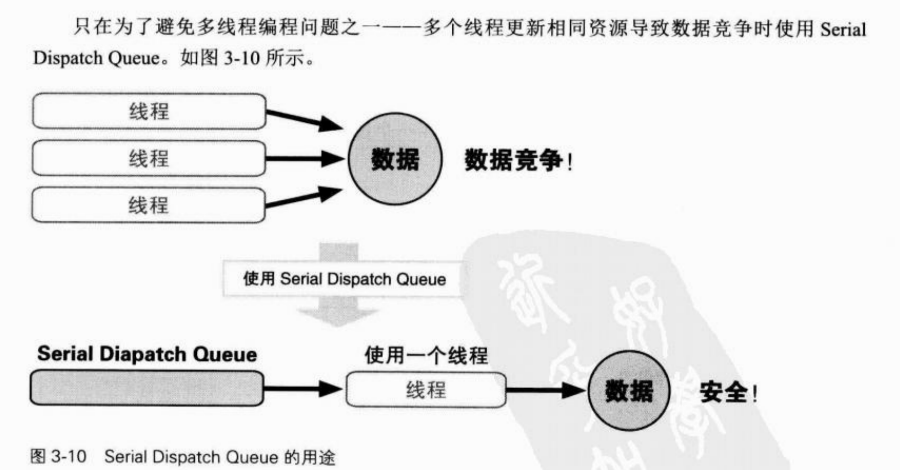dispatch_queue_create（）

dispatch_queue_create 函数的返回值为表示Dispatch Queue的“dispatch_queue_t类型”

### 4.2.3 Main Dispatch Queue/Global Dispatch Queue

Main Dispatch Queue 是在主线程中执行的Dispatch Queue，因为主线程只有一个，所以Main Dispatch Queue也就是Serial Dispatch Queue。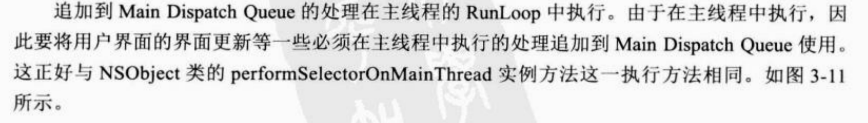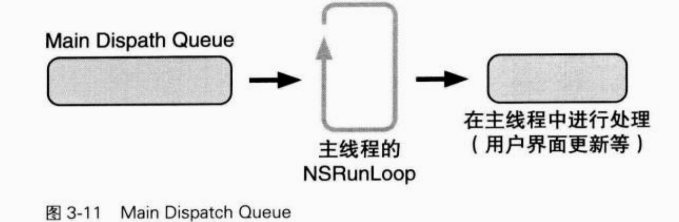Global Dispatch Queue是所有应用程序都能使用的Concurrent Dispatch Queue。没必要通过dispatch_queue_create 函数逐个生成Concurrent Dispatch Queue。只要获取Global Dispatch Queue使用即可。

Global Dispatch Queue有四个执行优先级。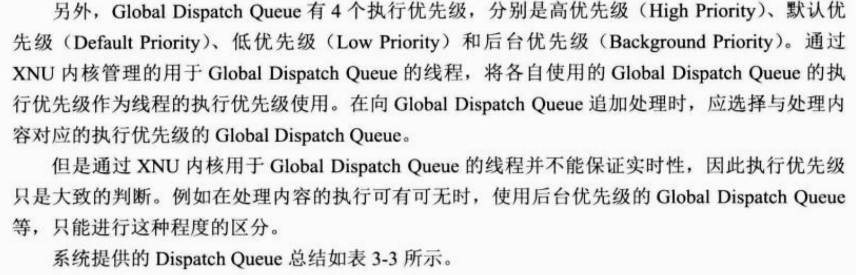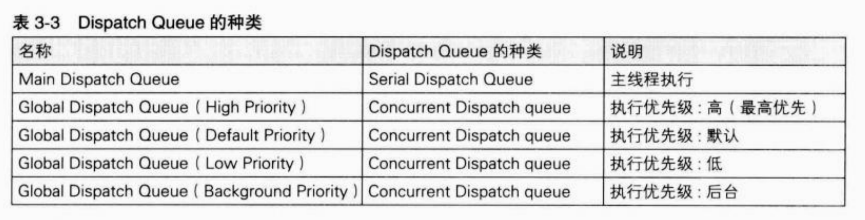``````Main Dispatch Queue 的获取方法
dispatch_queue_t mainDispatchQueue = dispatch_get_main_queue();
Global Dispatch Queue （高优先级）的获取方法
dispatch_queue_t globalDispatchQueueHigh = dispatch_get_global_queue(DISPATCH_QUEUE_PRIORITY_HIGH, 0);
Global Dispatch Queue （默认优先级）的获取方法
dispatch_queue_t globalDispatchQueueDefault = dispatch_get_global_queue(DISPATCH_QUEUE_PRIORITY_DEFAULT, 0);
Global Dispatch Queue （低优先级）的获取方法
dispatch_queue_t globalDispatchQueueLow = dispatch_get_global_queue(DISPATCH_QUEUE_PRIORITY_Low, 0);
Global Dispatch Queue （默认优先级）的获取方法
dispatch_queue_t globalDispatchQueueBackground = dispatch_get_global_queue(DISPATCH_QUEUE_PRIORITY_BACKGROUND, 0);``````

### 4.2.4 dispatch_set_ target_queue

dispatch_queue_create函数生成的Dispatch Queue 不管是Serial Dispatch Queue还是Concurrent Dispatch Queue，都与默认优先级Global Dispatch Queue相同执行优先级的线程。而变更生成的Dispatch Queue的执行优先级要使用dispatch_set_ target_queue函数。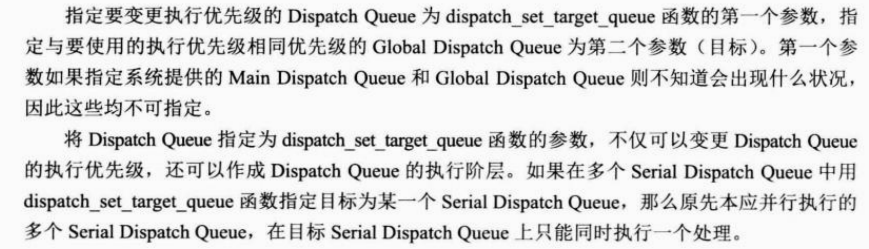### 4.2.4 dispatch_after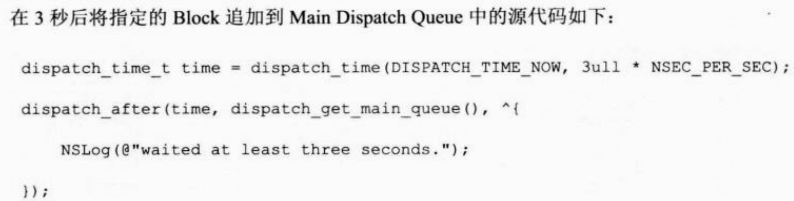dispatch_time函数能够获取从第一个参数dispatch_time_t类型值中指定的时间开始，到第二个参数指定的毫微秒单位时间后的时间。第一次参数经常使用的值是之前源代码中出现的DISPATCH_TIME_NOW 表示现在的时间。

``````// 表示从现在开始一秒后的dispatch_time_t 类型的值
dispatch_time_t time = dispatch_time(DISPATCH_TIME_NOW, lull * NSEC_PER_SEC);

// 表示从现在开始150毫秒后的dispatch_time_t 类型的值
dispatch_time_t time = dispatch_time(DISPATCH_TIME_NOW, 120lull * NSEC_PER_SEC);``````

### Tensorflow的安装与配置

Tensorflow的安装与配置 经过多次的安装失败&#xff0c;找寻原因&#xff0c;最终安装成功。 1、基于之前安装的anaconda的基础上进行tensorflow的安装&#xff0c;首先通过按windows键和R键弹出运行框&#xff0c;输入CMD回车&#xff0c;进入cmd命令窗口&#xff0c;先查询…

### 基本任务1.4java异常捕捉机制

Java异常捕捉机制 任务要求&#xff1a; 一、完成一个 java application应用程序&#xff0c;完成ca/b 的计算并输出c的结果&#xff0c;可以为a和b在程序中赋初值、或者接收用户通过键盘输入a和b的数值文本后转换为数字等&#xff0c;在程序要求当 b 为0时c的计算结果正确。…